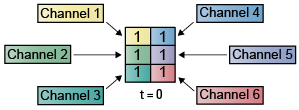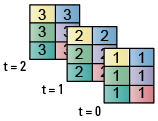## Import and Export Signals for Sample-Based Processing

In sample-based processing, blocks process signals one sample at a time. Each element of the input signal represents one sample in a distinct channel. For example, from a sample-based processing perspective, the following 3-by-2 matrix contains the first sample in each of six independent channels.When you configure a block to perform sample-based processing, the block interprets scalar input as a single-channel signal. Similarly, the block interprets an M-by-N matrix as multichannel signal with M*N independent channels. For example, in sample-based processing, blocks interpret the following sequence of 3-by-2 matrices as a six-channel signal.For more information about the recent changes to frame-based processing, see the Frame-based processing changes section of the DSP System Toolbox™ Release Notes.

### Import Vector Signals for Sample-Based Processing

The Signal From Workspace block generates a vector signal for sample-based processing when the variable or expression in the Signal parameter is a matrix and the Samples per frame parameter is set to `1`. Each column of the input matrix represents a different channel. Beginning with the first row of the matrix, the block outputs one row of the matrix at each sample time. Therefore, if the Signal parameter specifies an M-by-N matrix, the output of the Signal From Workspace block is M 1-by-N row vectors representing N channels.

The figure below is a graphical representation of this process for a 6-by-4 workspace matrix, `A`.In the following example, you use the Signal From Workspace block to import the vector signal into your model.

1. Open the Signal From Workspace Example 3 model by typing `ex_importsbvectorsigs` at the MATLAB® command line.2. At the MATLAB command line, type ```A = [1:100;-1:-1:-100]';```

The matrix `A` represents a two column signal, where each column is a different channel.

3. At the MATLAB command line, type B = 5*ones(100,1);

The vector `B` represents a single-channel signal.

4. Double-click the Signal From Workspace block, and set the block parameters as follows:

• Signal = ```[A B]```

• Sample time = `1`

• Samples per frame = `1`

• Form output after final data value = `Setting to zero`

The Signal expression `[A B]` uses the standard MATLAB syntax for horizontally concatenating matrices and appends column vector `B` to the right of matrix `A`. The Signal From Workspace block outputs a signal with a sample period of 1 second. After the block has output the signal, all subsequent outputs have a value of zero.

5. Save these parameters and close the dialog box by clicking .

6. Run the model.

The following figure is a graphical representation of the model's behavior during simulation.The first row of the input matrix [`A B]` is output at time `t=0`, the second row of the input matrix is output at time `t=1`, and so on.

You have now successfully imported a vector signal with three channels into your signal processing model using the Signal From Workspace block.

### Import Matrix Signals for Sample-Based Processing

The Signal From Workspace block generates a matrix signal that is convenient for sample-based processing. Beginning with the first page of the array, the block outputs a single page of the array to the output at each sample time. Therefore, if the Signal parameter specifies an M-by-N-by-P array, the output of the Signal From Workspace block is P M-by-N matrices representing M*N channels. The block receiving this signal does sample-based processing or frame-based processing on the signal based on the parameters set in the block dialog box.

The following figure is a graphical illustration of this process for a 6-by-4-by-5 workspace array `A`.In the following example, you use the Signal From Workspace block to import a four-channel matrix signal into a Simulink® model.

1. Open the Signal From Workspace Example 4 model by typing `ex_importsbmatrixsigs` at the MATLAB command line.Also, the following variables are loaded into the MATLAB workspace:

 `Fs` `1x1` `8` `double array` `dsp_examples_A` `2x2x100` `3200` `double array` `dsp_examples_sig1` `1x1x100` `800` `double array` `dsp_examples_sig12` `1x2x100` `1600` `double array` `dsp_examples_sig2` `1x1x100` `800` `double array` `dsp_examples_sig3` `1x1x100` `800` `double array` `dsp_examples_sig34 ` `1x2x100` `1600` `double array` `dsp_examples_sig4 ` `1x1x100` `800` `double array` `mtlb` `4001x1` `32008` `double array`
2. Double-click the Signal From Workspace block. Set the block parameters as follows, and then click :

• Signal = `dsp_examples_A`

• Sample time = `1`

• Samples per frame = `1`

• Form output after final data value = `Setting to zero`

The `dsp_examples_A` array represents a four-channel signal with 100 samples in each channel. This is the signal that you want to import, and it was created in the following way:

```dsp_examples_sig1 = reshape(1:100,[1 1 100]) dsp_examples_sig2 = reshape(-1:-1:-100,[1 1 100]) dsp_examples_sig3 = zeros(1,1,100) dsp_examples_sig4 = 5*ones(1,1,100) dsp_examples_sig12 = cat(2,sig1,sig2) dsp_examples_sig34 = cat(2,sig3,sig4) dsp_examples_A = cat(1,sig12,sig34) % 2-by-2-by-100 array ```
3. Run the model.

The figure below is a graphical representation of the model's behavior during simulation.The Signal From Workspace block imports the four-channel signal from the MATLAB workspace into the Simulink model one matrix at a time.

You have now successfully imported a 4-channel matrix signal into your model using the Signal From Workspace block.

### Export Signals for Sample-Based Processing

The To Workspace (Simulink) and Triggered To Workspace blocks are the primary blocks for exporting signals of all dimensions from a Simulink model to the MATLAB workspace.

A signal with M*N channels, is represented in Simulink as a sequence of M-by-N matrices. When the input to the To Workspace block is a signal created for sample-based processing, the block creates an M-by-N-by-P array in the MATLAB workspace containing the P most recent samples from each channel. The number of pages, P, is specified by the Limit data points to last parameter. The newest samples are added at the end of the array.

The following figure is the graphical illustration of this process using a 6-by-4 signal exported to workspace array `A`.The workspace array always has time running along its third dimension, P. Samples are saved along the P dimension whether the input is a matrix, vector, or scalar (single channel case).

In the following example you use a To Workspace block to export a matrix signal to the MATLAB workspace.

1. Open the Signal From Workspace Example 6 model by typing `ex_exportsbsigs` at the MATLAB command line.Also, the following variables are loaded into the MATLAB workspace:

 `dsp_examples_A` `2x2x100` `3200` `double array` `dsp_examples_sig1` `1x1x100` `800` `double array` `dsp_examples_sig12` `1x2x100` `1600` `double array` `dsp_examples_sig2` `1x1x100` `800` `double array` `dsp_examples_sig3` `1x1x100` `800` `double array` `dsp_examples_sig34` `1x2x100` `1600` `double array` `dsp_examples_sig4` `1x1x100` `800` `double array`

In this model, the Signal From Workspace block imports a four-channel matrix signal called `dsp_examples_A`. This signal is then exported to the MATLAB workspace using a To Workspace block.

2. Double-click the Signal From Workspace block. Set the block parameters as follows, and then click :

• Signal = `dsp_examples_A`

• Sample time = `1`

• Samples per frame = `1`

• Form output after final data value = `Setting to zero`

Based on these parameters, the Signal From Workspace block outputs a signal with a sample period of 1 second. After the block has output the signal, all subsequent outputs have a value of zero.

3. Double-click the To Workspace block. Set the block parameters as follows, and then click :

• Variable name = `dsp_examples_yout`

• Limit data points to last parameter to `inf`

• Decimation = `1`

Based on these parameters, the To Workspace block exports its input signal to a variable called `dsp_examples_yout` in the MATLAB workspace. The workspace variable can grow indefinitely large in order to capture all of the input data. The signal is not decimated before it is exported to the MATLAB workspace.

4. Run the model.

5. At the MATLAB command line, type `dsp_examples_yout`.

The four-channel matrix signal, `dsp_examples_A`, is output at the MATLAB command line. The following is a portion of the output that is displayed.

```dsp_examples_yout(:,:,1) = 1 -1 0 5 dsp_examples_yout(:,:,2) = 2 -2 0 5 dsp_examples_yout(:,:,3) = 3 -3 0 5 dsp_examples_yout(:,:,4) = 4 -4 0 5 ```

Each page of the output represents a different sample time, and each element of the matrices is in a separate channel.

You have now successfully exported a four-channel matrix signal from a Simulink model to the MATLAB workspace using the To Workspace block.